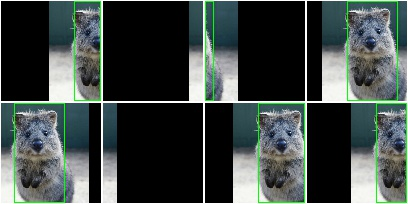# augmenters.meta¶

## Sequential¶

List augmenter that may contain other augmenters to apply in sequence or random order.

API link: `Sequential`

Example. Apply in predefined order:

```import imgaug.augmenters as iaa
aug = iaa.Sequential([
iaa.Affine(translate_px={"x":-40}),
])
```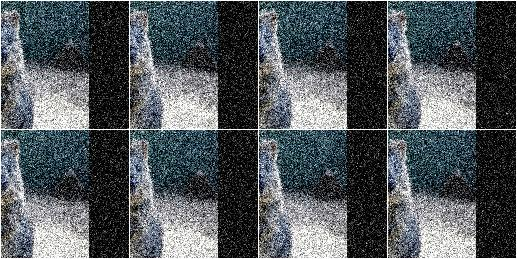Example. Apply in random order (note that the order is sampled once per batch and then the same for all images within the batch):

```aug = iaa.Sequential([
iaa.Affine(translate_px={"x":-40}),
], random_order=True)
```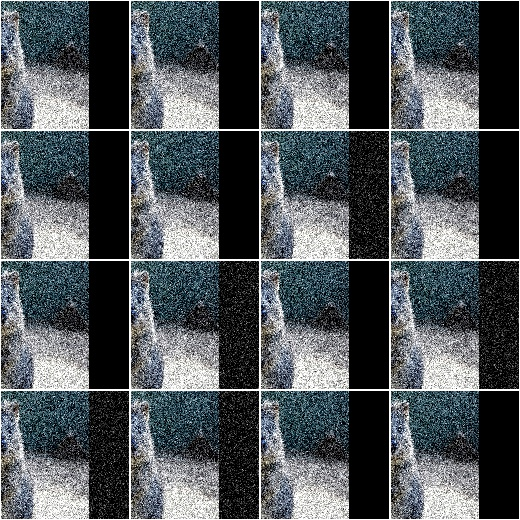## SomeOf¶

List augmenter that applies only some of its children to images.

API link: `SomeOf`

Example. Apply two of four given augmenters:

```import imgaug.augmenters as iaa
aug = iaa.SomeOf(2, [
iaa.Affine(rotate=45),
iaa.Sharpen(alpha=0.5)
])
```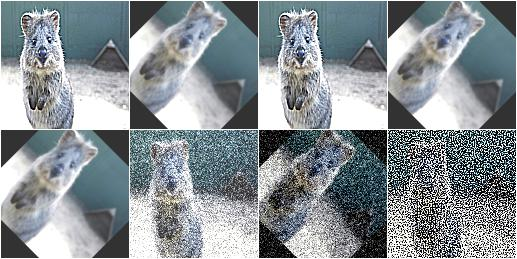Example. Apply `0` to `<max>` given augmenters (where `<max>` is automatically replaced with the number of children):

```aug = iaa.SomeOf((0, None), [
iaa.Affine(rotate=45),
iaa.Sharpen(alpha=0.5)
])
```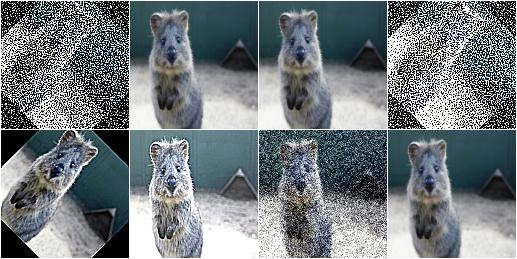Example. Pick two of four given augmenters and apply them in random order:

```aug = iaa.SomeOf(2, [
iaa.Affine(rotate=45),
iaa.Sharpen(alpha=0.5)
], random_order=True)
```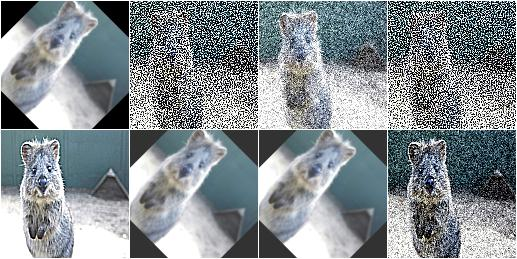## OneOf¶

Augmenter that always executes exactly one of its children.

API link: `OneOf()`

Example. Apply one of four augmenters to each image:

```import imgaug.augmenters as iaa
aug = iaa.OneOf([
iaa.Affine(rotate=45),
iaa.Sharpen(alpha=0.5)
])
```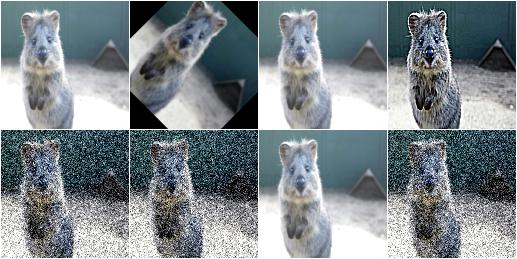## Sometimes¶

Augment only p percent of all images with one or more augmenters.

API link: `Sometimes`

Example. Apply gaussian blur to about 50% of all images:

```import imgaug.augmenters as iaa
aug = iaa.Sometimes(0.5, iaa.GaussianBlur(sigma=2.0))
```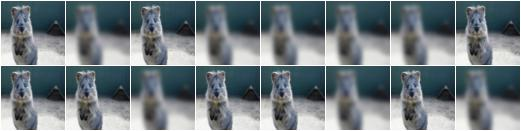Example. Apply gaussian blur to about 50% of all images. Apply a mixture of affine rotations and sharpening to the other 50%.

```aug = iaa.Sometimes(
0.5,
iaa.GaussianBlur(sigma=2.0),
iaa.Sequential([iaa.Affine(rotate=45), iaa.Sharpen(alpha=1.0)])
)
```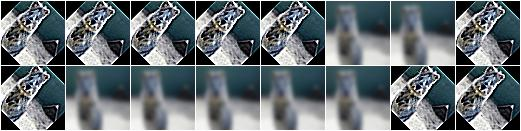## WithChannels¶

Apply child augmenters to specific channels.

API link: `WithChannels`

Example. Increase each pixel’s R-value (redness) by `10` to `100`:

```import imgaug.augmenters as iaa
```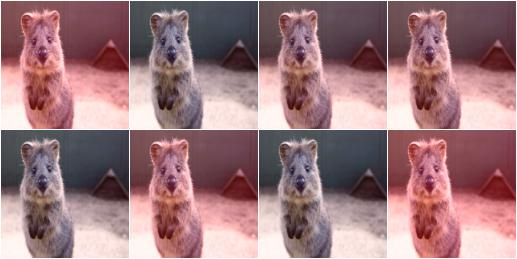Example. Rotate each image’s red channel by `0` to `45` degrees:

```aug = iaa.WithChannels(0, iaa.Affine(rotate=(0, 45)))
```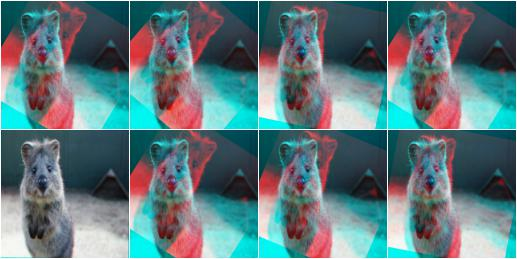## Identity¶

Augmenter that does not change the input data.

This augmenter is useful e.g. during validation/testing as it allows to re-use the training code without actually performing any augmentation.

API link: `Identity`

Example. Create an augmenter that does not change inputs:

```import imgaug.augmenters as iaa
aug = iaa.Identity()
```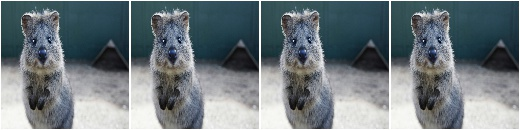## Noop¶

Alias for augmenter `Identity`.

It is recommended to now use `Identity`. `Noop` might be deprecated in the future.

API link: `Noop`

Example. Create an augmenter that does nothing:

```import imgaug.augmenters as iaa
aug = iaa.Noop()
```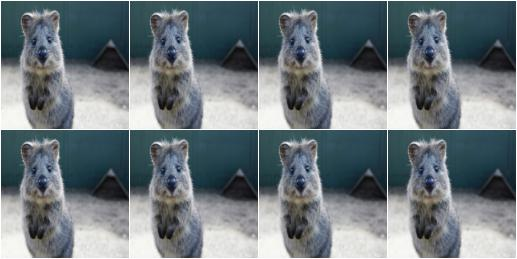## Lambda¶

Augmenter that calls a lambda function for each batch of input image.

API link: `Lambda`

Example. Replace in every image each fourth row with black pixels:

```import imgaug.augmenters as iaa

def img_func(images, random_state, parents, hooks):
for img in images:
img[::4] = 0
return images

def keypoint_func(keypoints_on_images, random_state, parents, hooks):
return keypoints_on_images

aug = iaa.Lambda(img_func, keypoint_func)
```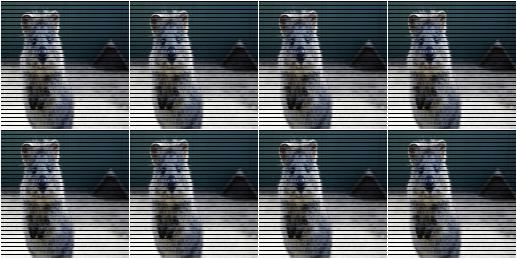## AssertLambda¶

Augmenter that runs an assert on each batch of input images using a lambda function as condition.

API link: `AssertLambda`

TODO examples

## AssertShape¶

Augmenter to make assumptions about the shape of input image(s) and keypoints.

API link: `AssertShape`

Example. Check if each image in a batch has shape `32x32x3`, otherwise raise an exception:

```import imgaug.augmenters as iaa
seq = iaa.Sequential([
iaa.AssertShape((None, 32, 32, 3)),
iaa.Fliplr(0.5) # only executed if shape matches
])
```

Example. Check if each image in a batch has a height in the range `32<=x<64`, a width of exactly `64` and either `1` or `3` channels:

```seq = iaa.Sequential([
iaa.AssertShape((None, (32, 64), 32, [1, 3])),
iaa.Fliplr(0.5)
])
```

## ChannelShuffle¶

Randomize the order of channels in input images.

API link: `ChannelShuffle`

Example. Shuffle all channels of 35% of all images:

```import imgaug.augmenters as iaa
aug = iaa.ChannelShuffle(0.35)
```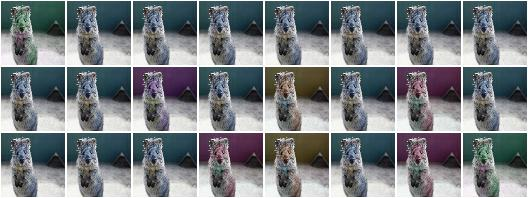Example. Shuffle only channels `0` and `1` of 35% of all images. As the new channel orders `0, 1` and `1, 0` are both valid outcomes of the shuffling, it means that for `0.35 * 0.5 = 0.175` or 17.5% of all images the order of channels `0` and `1` is inverted.

```aug = iaa.ChannelShuffle(0.35, channels=[0, 1])
```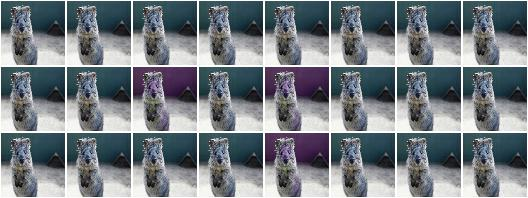## RemoveCBAsByOutOfImageFraction¶

Remove coordinate-based augmentables exceeding an out of image fraction.

This augmenter inspects all coordinate-based augmentables (e.g. bounding boxes, line strings) within a given batch and removes any such augmentable which’s out of image fraction is exactly a given value or greater than that. The out of image fraction denotes the fraction of the augmentable’s area that is outside of the image, e.g. for a bounding box that has half of its area outside of the image it would be `0.5`.

Example. Translate all inputs by `-100` to `100` pixels on the x-axis, then remove any coordinate-based augmentable (e.g. bounding boxes) which has at least `50%` of its area outside of the image plane:

```import imgaug.augmenters as iaa
aug = iaa.Sequential([
iaa.Affine(translate_px={"x": (-100, 100)}),
iaa.RemoveCBAsByOutOfImageFraction(0.5)
])
```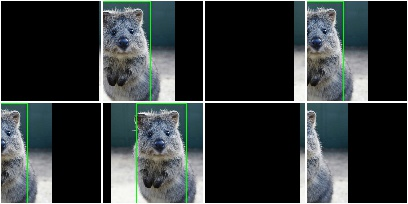Example. Create a bounding box on an example image, then translate the image so that `50%` of the bounding box’s area is outside of the image and compare the effects and using `RemoveCBAsByOutOfImageFraction` with not using it.

```import imgaug as ia
import imgaug.augmenters as iaa
image = ia.quokka_square((100, 100))
bb = ia.BoundingBox(x1=50-25, y1=0, x2=50+25, y2=100)
bbsoi = ia.BoundingBoxesOnImage([bb], shape=image.shape)
aug_without = iaa.Affine(translate_px={"x": 51})
aug_with = iaa.Sequential([
iaa.Affine(translate_px={"x": 51}),
iaa.RemoveCBAsByOutOfImageFraction(0.5)
])

image_without, bbsoi_without = aug_without(
image=image, bounding_boxes=bbsoi)
image_with, bbsoi_with = aug_with(
image=image, bounding_boxes=bbsoi)

assert len(bbsoi_without.bounding_boxes) == 1
assert len(bbsoi_with.bounding_boxes) == 0
```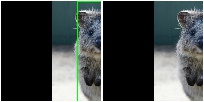## ClipCBAsToImagePlanes¶

Clip coordinate-based augmentables to areas within the image plane.

This augmenter inspects all coordinate-based augmentables (e.g. bounding boxes, line strings) within a given batch and from each of them parts that are outside of the image plane. Parts within the image plane will be retained. This may e.g. shrink down bounding boxes. For keypoints, it removes any single points outside of the image plane. Any augmentable that is completely outside of the image plane will be removed.

API link: `ClipCBAsToImagePlanes`

Example. Translate input data on the x-axis by `-100` to `100` pixels, then cut all coordinate-based augmentables (e.g. bounding boxes) down to areas that are within the image planes of their corresponding images:

```import imgaug.augmenters as iaa
aug = iaa.Sequential([
iaa.Affine(translate_px={"x": (-100, 100)}),
iaa.ClipCBAsToImagePlanes()
])
```